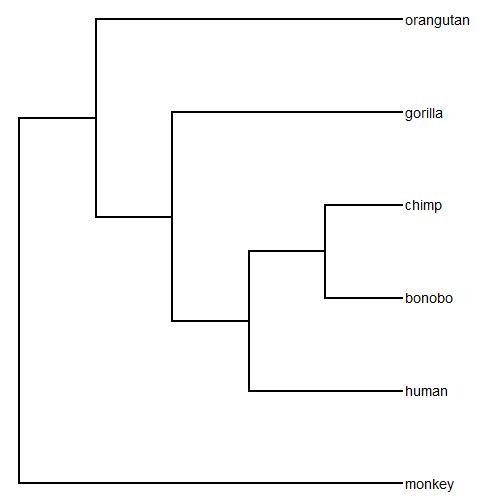## Monday, January 30, 2017

### Creating a phylogenetic tree in R using `phytools::bind.tip` in interactive mode

I just made a short video showing how to draw a tree “free-hand” in R using the `interactive=TRUE` mode of the phytools function `bind.tip`.

The video is here:

The syntax for this exercise (in case it cannot be easily read from the video) is as follows:

``````## first load our packages:
library(phytools)

## next create a two taxon tree that subtends the root:
tree<-pbtree(n=2,tip.label=c("monkey","human"))

## now interactively bind the addt'l tips we want onto this tree:
tips<-c("bonobo","chimp","gorilla","orangutan")
for(i in 1:length(tips)) tree<-bind.tip(tree,tips[i],interactive=TRUE)

## strip edge lengths (here they were arbitrary):
tree\$edge.length<-NULL

## plot our tree:
roundPhylogram(tree)

## done.
``````

The objected created in memory is just a regular `"phylo"` class object, so it can be plotted:

``````plotTree(tree,fsize=1.2)
``````written to file:

``````write.tree(tree)
``````
``````##  "(monkey,(((human,(bonobo,chimp)),gorilla),orangutan));"
``````

etc.

In a subsequent post I will demonstrate how to add edge lengths based on divergence dates associated with individual nodes of the tree.